StatLect

# Interval estimation

Interval estimation (or set estimation) is a kind of statistical inference in which we search for an interval of values that contains the true parameter with high probability. Such an interval is called a confidence interval.## The mathematical framework

The mathematical framework of interval estimation is the same of other statistical inference methods:

• a sample is employed to make statements about the probability distribution from which the sample has been generated;

• the samplecan be regarded as a realization of a random vector, whose joint distribution function, denoted by, is unknown;

•is assumed to belong to a set of distribution functions, called statistical model.

Furthermore, the model is parametrized:

• in a parametric model, the setis put into correspondence with a set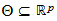of-dimensional real vectors;

•is called the parameter space and its elements are called parameters;

• there is a true parameter, which is associated with the unknown data-generating distribution•is assumed to be unique (this is not strictly necessary, but we do it to simplify the exposition).

## Confidence set

In set estimation, the aim is to choose a subsetin such a way thathas a high probability of containing the unknown parameter.

The chosen subsetis called a set estimate ofor a confidence set for.

## Confidence interval

A special terminology is used in the case in which:

1. the parameter spaceis a subset of the set of real numbers;

2. the subsetis chosen among the intervals of(e.g. intervals of the kind)

In this case, we speak about:

• interval estimation (instead of set estimation);

• interval estimate (instead of set estimate);

• confidence interval (instead of confidence set).

## Interval estimator

When the set estimateis produced using a predefined rule (a function) that associates a set estimateto eachin the support of, we can write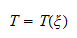The functionis called a set estimator (interval estimator).

## Coverage probability

The probability that the interval estimatorcontains the true parameter is called coverage probability.

In formal terms, the coverage probability of an interval estimator is defined as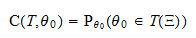where the notationis used to indicate that the probability is calculated using the distribution function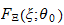associated to the true parameter.

In this definition, the random quantity is the interval, while the parameteris fixed.

## How the coverage probability is chosen

The coverage probability is usually chosen by the statistician.

Intuitively, before observing the data the statistician makes a statement: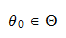whereis the parameter space, containing all the parameters that are deemed plausible.

The statistician believes the statement to be true, but the statement is not very informative becauseis a very large set.

After observing the data, she makes a more informative statement:This statement is more informative becauseis smaller than, but it has a positive probability of being wrong (which is the complement toof the coverage probability).

In controlling this probability, the statistician faces a trade-off: if she decreases the probability of being wrong, then her statements become less informative; on the contrary, if she increases the probability of being wrong, then her statements become more informative.

## Level of confidence

In practice, the coverage probability is seldom known because it depends on the unknown parameter(although in some important cases it is equal for all parameters belonging to the parameter space).

When the coverage probability is not known, it is customary to compute the confidence coefficient, which is defined as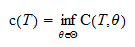In other words, the confidence coefficientis equal to the smallest possible coverage probability.

The confidence coefficient is also often called level of confidence.

## Size of the confidence set

We already mentioned that there is a trade-off in the construction and choice of a set estimator.

On the one hand, we want our set estimatorto have a high coverage probability, that is, we want the setto include the true parameter with a high probability.

On the other hand, we want the size ofto be as small as possible, so as to make our interval estimate more precise.

What do we mean by size of?

If the parameter spaceis unidimensional andis an interval estimate, then the size ofis its length.

If the spaceis multidimensional, then the size ofis its volume.

The size of a confidence set is also called measure of a confidence set. For those who have a grasp of measure theory, the name stems from the fact that Lebesgue measure is the generalization of volume in multidimensional spaces.

## Expected size of the confidence set

If we denote bythe size of a confidence set, then we can also define the expected size of a set estimator: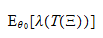where the notationis used to indicate that the expected value is calculated using the true distribution function.

Like the coverage probability, also the expected size of a set estimator depends on the unknown parameter.

Hence, unless it is a constant function of, one needs to somehow estimate it or to take the infimum over all possible values of the parameter, as we did above for coverage probabilities.

## Other criteria to evaluate set estimators

Although size is probably the simplest criterion to evaluate and select interval estimators, there are several other criteria. We do not discuss them here, but we refer the reader to the very nice exposition in Berger and Casella (2002).

## Examples

Examples of interval estimation problems can be found in the following lectures:

## References

Berger, R. L. and G. Casella (2002) "Statistical inference", Duxbury Advanced Series.